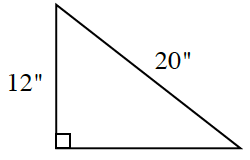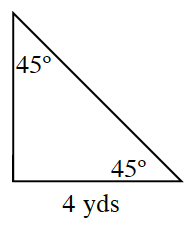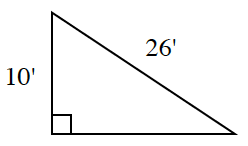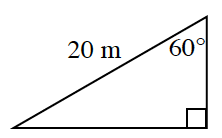### Home > CCG > Chapter 5 > Lesson 5.2.2 > Problem5-64

5-64.

The sides of each of the triangles below can be found using one of the shortcuts from Section 5.2. Try to find the missing lengths using your patterns. Do not use a calculator.

1.Is this triangle similar to a Pythagorean Triple?

The triangle is similar to the Pythagorean Triple $\text{3-4-5}$, with a scale factor of $4$.

The missing side is similar to $4$ on the $\text{3-4-5}$ triangle.
With a scale factor of $4$, the missing side is $4·4=16$ inches.

1.This is a $45^\circ\text{-}45^\circ\text{-}90^\circ$ triangle. What is the scale factor between this
$45^\circ\text{-}45^\circ\text{-}90^\circ$ special triangle and the given triangle?

With a scale factor of $4$. Missing sides are...

$4$ and $4\sqrt{2}$.

1.Is this triangle similar to a Pythagorean Triple of $\text{3-4-5}$ or $\text{5-12-13}$?

1.With a $30^\circ\text{-}60^\circ\text{-}90^\circ$ triangle, the short side is half the length of the hypotenuse so it is $10$.
The side opposite the $60^\circ$ angle is multiplied by the square root of $3$. So it is...

$10\sqrt{3} \text{ m}$